# Solution assignment 07 Optimizing f(x)

### Assignment 7

Determine the extremes of the function: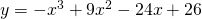Verify in which point there is a maximum or a minimum by using the second derivative.

### Solution

We find the candidates for extremes by calculating the first derivative and making it equal to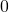: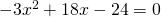or: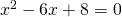and this equation has the solutions: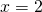or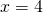The second derivative is: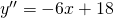Forwe have: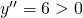and thus there is a minimum forForwe have: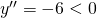and thus there is a maximum for.

0